Ask your WordPress questions! Pay money and get answers fast!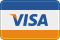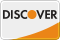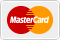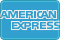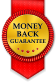# Gravity Forms - Populate Checkbox in Form from Multiple Single Line Text Input Answers from User WordPress

• SOLVED

Hello,

This is a Gravity Forms question/problem. I have multi-page form where I need checkboxes later in the form to be populated with the user's answers from single line text inputs earlier in the form. To simplify it:

// Page One Inputs

Single Line Text Input : input_1
Single Line Text Input : input_2
Single Line Text Input : input _3
Single Line Text Input : input _4

// Then on another page in the form

Checkbox Field 1
option 1 : input_1
option 2 : input_2
option 3 : input_3
option 4 : input_4

// Another page in the form

Checkbox Field 2
option 1 : input_1
option 2 : input_2
option 3 : input_3
option 4 : input_4

The tricky part is that the checkbox is built from MULTIPLE single line text inputs, so it's many-to-one in its relationship.

Thanks!

2018-03-08

Try this, note there are some IDs you need to update for your form

```add_filter( 'gform_pre_render_1', 'my_populate_checkbox' );//Change the 1 to your form ID function my_populate_checkbox( \$form ) { \$choices = array(); \$single_text_ids = array( 1, 2, 3, 4); //text input IDs foreach( \$single_text_ids as \$id ){ \$choices[] = ('text' => rgpost( 'input_1_'.\$id ), 'value' => rgpost( 'input_1_'.\$id ) ); //The ones need to be form ID } foreach( \$form['fields'] as &\$field ) { if( 1 === \$field->id ) { //Change to your checkbox ID \$field['choices'] = \$choices; } } return \$form; }```

Wait, you need to check for page, try this:

```add_filter( 'gform_pre_render_1', 'my_populate_checkbox' );//Change the 1 to your form ID function my_populate_checkbox( \$form ) { if (!is_admin()) { \$current_page = rgpost('gform_source_page_number_' . \$form['id']) ? rgpost('gform_source_page_number_' . \$form['id']) : 1; if (\$current_page == 10) { //Change to page of checkbox \$choices = array(); \$single_text_ids = array( 1, 2, 3, 4); //text input IDs foreach( \$single_text_ids as \$id ){ \$choices[] = ('text' => rgpost( 'input_1_'.\$id ), 'value' => rgpost( 'input_1_'.\$id ) ); //The ones need to be form ID } foreach( \$form['fields'] as &\$field ) { if( 1 === \$field->id ) { //Change to your checkbox ID \$field['choices'] = \$choices; } } } } return \$form; }```

Kyle, I'm getting a syntax error for the \$choices array on line 12. Thoughts?

I was missing array text, try this

```add_filter( 'gform_pre_render_1', 'my_populate_checkbox' );//Change the 1 to your form ID function my_populate_checkbox( \$form ) { if (!is_admin()) { \$current_page = rgpost('gform_source_page_number_' . \$form['id']) ? rgpost('gform_source_page_number_' . \$form['id']) : 1; if (\$current_page == 10) { //Change to page of checkbox \$choices = array(); \$single_text_ids = array( 1, 2, 3, 4); //text input IDs foreach( \$single_text_ids as \$id ){ \$choices[] = array('text' => rgpost( 'input_1_'.\$id ), 'value' => rgpost( 'input_1_'.\$id ) ); //The ones need to be form ID } foreach( \$form['fields'] as &\$field ) { if( 1 === \$field->id ) { //Change to your checkbox ID \$field['choices'] = \$choices; } } } } return \$form; }```

Not working for me. Replaced all form IDs, added the input(s) to the array, changed the field ID of the checkbox. I'm using a test form with just two pages, so I changed the page ID to page 2. When I get to the Checkbox, it just shows the one default checkbox option that you have to leave in by default--no added items from the input.

Can you paste your updated code with the inputs. Also, try setting page to 1 instead of 2, it might start at zero.

Changed ID to 1 instead of 2, and now I'm getting two blank checkbox items...so closer.

```add_filter( 'gform_pre_render_20', 'my_populate_checkbox' );//Change the 1 to your form ID function my_populate_checkbox( \$form ) { if (!is_admin()) { \$current_page = rgpost('gform_source_page_number_' . \$form['id']) ? rgpost('gform_source_page_number_' . \$form['id']) : 1; if (\$current_page == 1) { //Change to page of checkbox \$choices = array(); \$single_text_ids = array(13,14); //text input IDs foreach( \$single_text_ids as \$id ){ \$choices[] = array('text' => rgpost( 'input_20_'.\$id ), 'value' => rgpost( 'input_20_'.\$id ) ); //The ones need to be form ID } foreach( \$form['fields'] as &\$field ) { if( 11 === \$field->id ) { //Change to your checkbox ID \$field['choices'] = \$choices; } } } } return \$form; }```If you want to send me a quick message here https://blueskycoding.com/contact-us/ I can hop on your site and get this working for you

2018-03-08

``` // replace 1 with the form id add_filter( 'gform_pre_render_1', 'populate_checkboxes' ); function populate_checkboxes( \$form ) { // get the current page \$current_page = GFFormDisplay::get_current_page( \$form['id'] ); // replace 2 with the page where the first checkbox field will show if ( \$current_page == 2 ) { foreach ( \$form['fields'] as &\$field ) { //replace 5 with the id of the first checkbox field if ( \$field->id == 5 ) { \$field['choices'] = array(); \$field['choices'][] = array( 'text' => \$_POST['input_1'], 'value' => \$_POST['input_1'] ); \$field['choices'][] = array( 'text' => \$_POST['input_2'], 'value' => \$_POST['input_2'] ); \$field['choices'][] = array( 'text' => \$_POST['input_3'], 'value' => \$_POST['input_3'] ); \$field['choices'][] = array( 'text' => \$_POST['input_4'], 'value' => \$_POST['input_4'] ); break; } } } // replace 3 with the page where the second checkbox field will show if ( \$current_page == 3 ) { foreach ( \$form['fields'] as &\$field ) { //replace 6 with the id of the second checkbox field if ( \$field->id == 6 ) { \$field['choices'] = array(); \$field['choices'][] = array( 'text' => \$_POST['input_1'], 'value' => \$_POST['input_1'] ); \$field['choices'][] = array( 'text' => \$_POST['input_2'], 'value' => \$_POST['input_2'] ); \$field['choices'][] = array( 'text' => \$_POST['input_3'], 'value' => \$_POST['input_3'] ); \$field['choices'][] = array( 'text' => \$_POST['input_4'], 'value' => \$_POST['input_4'] ); break; } } } return \$form; } ```